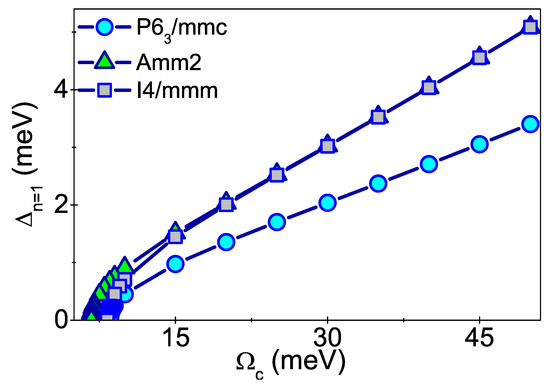Next Article in Journal
Conformal Symmetry, Accelerated Observers, and Nonlocality
Next Article in Special Issue
Quantum Behavior of a PT -Symmetric Two-Mode System with Cross-Kerr Nonlinearity
Previous Article in Journal
On Quasi-Homogeneous Production Functions

## Volume 11, Issue 8

Article MenuMetrics 0

## Export Article

Open AccessArticle

# Characteristics of the s–Wave Symmetry Superconducting State in the BaGe3 Compound

1
Division of Theoretical Physics, Institute of Physics, Jan Długosz University in Czȩstochowa, Ave. Armii Krajowej 13/15, 42-200 Czȩstochowa, Poland
2
Institute of Physics, Czȩstochowa University of Technology, Ave. Armii Krajowej 19, 42-200 Czȩstochowa, Poland
3
Faculty of Chemistry, University of Warsaw, Pasteura 1, 02-093 Warsaw, Poland
*
Author to whom correspondence should be addressed.
Symmetry 2019, 11(8), 977; https://doi.org/10.3390/sym11080977
Received: 29 June 2019 / Revised: 21 July 2019 / Accepted: 22 July 2019 / Published: 1 August 2019
(This article belongs to the Special Issue Quantum Information and Symmetry)
|
PDF [523 KB, uploaded 13 August 2019]
|

# Abstract

Thermodynamic properties of the s–wave symmetry superconducting phase in three selected structures of the $BaGe 3$ compound ( $P 6 3 / m m c$ , $A m m 2$ , and $I 4 / m m m$ ) were discussed in the context of DFT results obtained for the Eliashberg function. This compound may enable the implementation of systems for quantum information processing. Calculations were carried out within the Eliashberg formalism due to the fact that the electron–phonon coupling constant falls within the range $λ ∈ 0.73 , 0.86$ . The value of the Coulomb pseudopotential was assumed to be $0.122$ , in accordance with the experimental results. The value of the Coulomb pseudopotential was assumed to be $0.122$ , in accordance with the experimental results. The existence of the superconducting state of three different critical temperature values, namely, $4.0$ K, $4.5$ K and $5.5$ K, depending on the considered structure, was stated. We determined the differences in free energy ( $Δ F$ ) and specific heat ( $Δ C$ ) between the normal and the superconducting states, as well as the thermodynamic critical field ( $H c$ ) as a function of temperature. A drop in the $H c$ value to zero at the temperature of 4.0 K was observed for the $P 6 3 / m m c$ structure, which is in good accordance with the experimental data. Further, the values of the dimensionless thermodynamic parameters of the superconducting state were estimated as: $R Δ = 2 Δ ( 0 ) / k B T c ∈ { 3.68 , 3.8 , 3.8 }$ , $R C = Δ C ( T c ) / C N ( T c ) ∈ { 1.55 , 1.71 , 1.75 }$ , and $R H = T c C N ( T c ) / H c 2 ( 0 ) ∈ { 0.168 , 0.16 , 0.158 }$ , which are slightly different from the predictions of the Bardeen–Cooper–Schrieffer theory ( $[ R Δ ] B C S = 3.53$ , $[ R C ] B C S = 1.43$ , and $[ R H ] B C S = 0.168$ ). This is caused by the occurrence of small retardation and strong coupling effects. View Full-Text
FiguresFigure 1

This is an open access article distributed under the Creative Commons Attribution License which permits unrestricted use, distribution, and reproduction in any medium, provided the original work is properly cited (CC BY 4.0).# Share & Cite This Article

MDPI and ACS Style

Szewczyk, K.A.; Drzazga-Szczȩśniak, E.A.; Jarosik, M.W.; Szczȩśniak, K.M.; Binek, S.M. Characteristics of the s–Wave Symmetry Superconducting State in the BaGe3 Compound. Symmetry 2019, 11, 977.

Note that from the first issue of 2016, MDPI journals use article numbers instead of page numbers. See further details here.

1

# Comments

[Return to top]
Symmetry EISSN 2073-8994 Published by MDPI AG, Basel, Switzerland RSS E-Mail Table of Contents Alert
Back to Top